Main MRPT website > C++ reference for MRPT 1.5.6
mrpt::poses::CPose3DQuat Class Reference

Detailed Description

A class used to store a 3D pose as a translation (x,y,z) and a quaternion (qr,qx,qy,qz).

For a complete description of Points/Poses, see mrpt::poses::CPoseOrPoint, or refer to the 2D/3D Geometry tutorial in the wiki.

To access the translation use x(), y() and z(). To access the rotation, use CPose3DQuat::quat().

This class also behaves like a STL container, since it has begin(), end(), iterators, and can be accessed with the [] operator with indices running from 0 to 6 to access the [x y z qr qx qy qz] as if they were a vector. Thus, a CPose3DQuat can be used as a 7-vector anywhere the MRPT math functions expect any kind of vector.

This class and CPose3D are very similar, and they can be converted to the each other automatically via transformation constructors.

CPose3D (for a class based on a 4x4 matrix instead of a quaternion), mrpt::math::TPose3DQuat, mrpt::poses::CPose3DQuatPDF for a probabilistic version of this class, mrpt::math::CQuaternion, CPoseOrPoint

Definition at line 41 of file CPose3DQuat.h.

#include <mrpt/poses/CPose3DQuat.h>

Inheritance diagram for mrpt::poses::CPose3DQuat: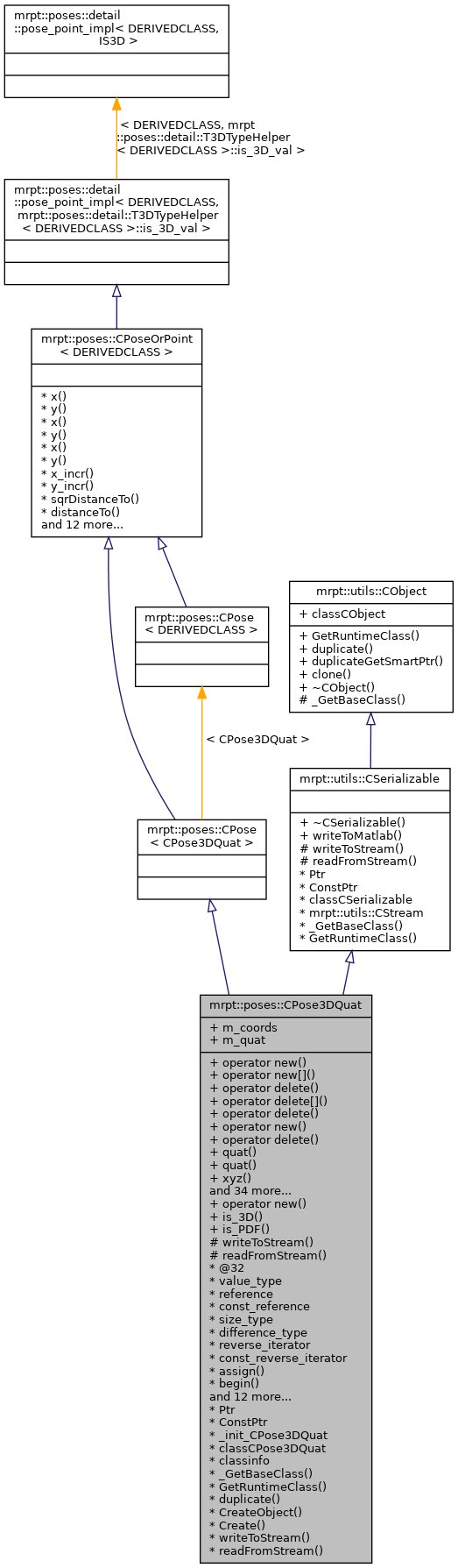Classes

struct  const_iterator

struct  iterator

Public Types

enum  { is_3D_val = 1 }

enum  { rotation_dimensions = 3 }

enum  { is_PDF_val = 1 }

typedef CPose3DQuat type_value
Used to emulate CPosePDF types, for example, in mrpt::graphs::CNetworkOfPoses. More...

typedef CPose3DQuat mrpt_autotype
See ops_containers.h. More...

Public Member Functions

voidoperator new (size_t size)

voidoperator new[] (size_t size)

void operator delete (void *ptr) throw ()

void operator delete[] (void *ptr) throw ()

void operator delete (void *memory, void *ptr) throw ()

voidoperator new (size_t size, const std::nothrow_t &) throw ()

void operator delete (void *ptr, const std::nothrow_t &) throw ()

mrpt::math::CQuaternionDoublequat ()

const
mrpt::math::CQuaternionDouble
quat () const

mrpt::math::CArrayDouble< 3 > & xyz ()

const mrpt::math::CArrayDouble< 3 > & xyz () const

CPose3DQuat ()
Default constructor, initialize translation to zeros and quaternion to no rotation. More...

CPose3DQuat (mrpt::math::TConstructorFlags_Quaternions)
Constructor which left all the quaternion members un-initialized, for use when speed is critical; Use UNINITIALIZED_POSE as argument to this constructor. More...

CPose3DQuat (TConstructorFlags_Poses)

CPose3DQuat (const double x, const double y, const double z, const mrpt::math::CQuaternionDouble &q)
Constructor with initilization of the pose - the quaternion is normalized to make sure it's unitary. More...

CPose3DQuat (const CPose3D &p)
Constructor from a CPose3D. More...

CPose3DQuat (const mrpt::math::TPose3DQuat &p)
Constructor from lightweight object. More...

CPose3DQuat (const mrpt::math::CMatrixDouble44 &M)
Constructor from a 4x4 homogeneous transformation matrix. More...

void getHomogeneousMatrix (mrpt::math::CMatrixDouble44 &out_HM) const
Returns the corresponding 4x4 homogeneous transformation matrix for the point(translation) or pose (translation+orientation). More...

void getAsVector (mrpt::math::CVectorDouble &v) const
Returns a 1x7 vector with [x y z qr qx qy qz]. More...

void getAsVector (mrpt::math::CArrayDouble< 7 > &v) const

void composeFrom (const CPose3DQuat &A, const CPose3DQuat &B)
Makes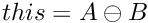this method is slightly more efficient than "this= A + B;" since it avoids the temporary object. More...

void inverseComposeFrom (const CPose3DQuat &A, const CPose3DQuat &B)
Makes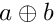this method is slightly more efficient than "this= A - B;" since it avoids the temporary object. More...

void composePoint (const double lx, const double ly, const double lz, double &gx, double &gy, double &gz, mrpt::math::CMatrixFixedNumeric< double, 3, 3 > *out_jacobian_df_dpoint=NULL, mrpt::math::CMatrixFixedNumeric< double, 3, 7 > *out_jacobian_df_dpose=NULL) const
Computes the 3D point G such as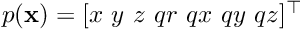. More...

void inverseComposePoint (const double gx, const double gy, const double gz, double &lx, double &ly, double &lz, mrpt::math::CMatrixFixedNumeric< double, 3, 3 > *out_jacobian_df_dpoint=NULL, mrpt::math::CMatrixFixedNumeric< double, 3, 7 > *out_jacobian_df_dpose=NULL) const
Computes the 3D point L such as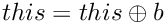. More...

template<class POINT1 , class POINT2 >
void composePoint (const POINT1 &L, POINT2 &G) const
Computes the 3D point G such as. More...

template<class POINT1 , class POINT2 >
void inverseComposePoint (const POINT1 &G, POINT2 &L) const
Computes the 3D point L such as. More...

CPoint3D operator+ (const CPoint3D &L) const
Computes the 3D point G such as. More...

mrpt::math::TPoint3D operator+ (const mrpt::math::TPoint3D &L) const
Computes the 3D point G such as. More...

virtual void operator*= (const double s)
Scalar multiplication (all x y z qr qx qy qz elements are multiplied by the scalar). More...

CPose3DQuatoperator+= (const CPose3DQuat &b)
Make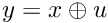. More...

CPose3DQuat operator+ (const CPose3DQuat &p) const
Return the composed pose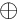. More...

CPose3DQuatoperator-= (const CPose3DQuat &b)
Make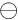. More...

CPose3DQuat operator- (const CPose3DQuat &p) const
Return the composed pose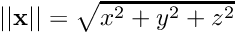. More...

void inverse ()
Convert this pose into its inverse, saving the result in itself. More...

void asString (std::string &s) const
Returns a human-readable textual representation of the object (eg: "[x y z qr qx qy qz]", angles in degrees.) More...

std::string asString () const

void fromString (const std::string &s)
Set the current object value from a string generated by 'asString' (eg: "[0.02 1.04 -0.8 1 0 0 0]" ) More...

const double & operator[] (unsigned int i) const

double & operator[] (unsigned int i)

void sphericalCoordinates (const mrpt::math::TPoint3D &point, double &out_range, double &out_yaw, double &out_pitch, mrpt::math::CMatrixFixedNumeric< double, 3, 3 > *out_jacob_dryp_dpoint=NULL, mrpt::math::CMatrixFixedNumeric< double, 3, 7 > *out_jacob_dryp_dpose=NULL) const
Computes the spherical coordinates of a 3D point as seen from the 6D pose specified by this object. More...

const type_valuegetPoseMean () const

type_valuegetPoseMean ()

void setToNaN () MRPT_OVERRIDE
Set all data fields to quiet NaN. More...

virtual mxArraywriteToMatlab () const
Introduces a pure virtual method responsible for writing to a mxArray Matlab object, typically a MATLAB struct whose contents are documented in each derived class. More...

mrpt::utils::CObjectPtr duplicateGetSmartPtr () const
Returns a copy of the object, indepently of its class, as a smart pointer (the newly created object will exist as long as any copy of this smart pointer). More...

CObjectclone () const
Cloning interface for smart pointers. More...

Static Public Member Functions

static voidoperator new (size_t size, void *ptr)

static bool is_3D ()

static bool is_PDF ()

Public Attributes

mrpt::math::CArrayDouble< 3 > m_coords
The translation vector [x,y,z]. More...

mrpt::math::CQuaternionDouble m_quat
The quaternion. More...

Static Public Attributes

static const
mrpt::utils::TRuntimeClassId
classCObject

RTTI stuff
static const
mrpt::utils::TRuntimeClassId
classCSerializable

Protected Member Functions

CSerializable virtual methods
void writeToStream (mrpt::utils::CStream &out, int *getVersion) const
Introduces a pure virtual method responsible for writing to a CStream. More...

void readFromStream (mrpt::utils::CStream &in, int version)
Introduces a pure virtual method responsible for loading from a CStream This can not be used directly be users, instead use "stream >> object;" for reading it from a stream or "stream >> object_ptr;" if the class is unknown apriori. More...

RTTI stuff

typedef CPose3DQuatPtr Ptr

typedef CPose3DQuatPtr ConstPtr

static mrpt::utils::CLASSINIT _init_CPose3DQuat

static mrpt::utils::TRuntimeClassId classCPose3DQuat

static const
mrpt::utils::TRuntimeClassId
classinfo

static const
mrpt::utils::TRuntimeClassId
_GetBaseClass ()

virtual const
mrpt::utils::TRuntimeClassId
GetRuntimeClass () const
Returns information about the class of an object in runtime. More...

virtual mrpt::utils::CObjectduplicate () const
Returns a copy of the object, indepently of its class. More...

static mrpt::utils::CObjectCreateObject ()

static CPose3DQuatPtr Create ()

STL-like methods and typedefs

enum  { static_size = 7 }

typedef double value_type
The type of the elements. More...

typedef double & reference

typedef const double & const_reference

typedef std::size_t size_type

typedef std::ptrdiff_t difference_type

typedef std::reverse_iterator
< iterator
reverse_iterator

typedef std::reverse_iterator
< const_iterator
const_reverse_iterator

void assign (const size_t N, const double val)

iterator begin ()

iterator end ()

const_iterator begin () const

const_iterator end () const

reverse_iterator rbegin ()

const_reverse_iterator rbegin () const

reverse_iterator rend ()

const_reverse_iterator rend () const

void swap (CPose3DQuat &o)

static size_type size ()

static bool empty ()

static size_type max_size ()

static void resize (const size_t n)

double x () const
Common members of all points & poses classes. More...

double & x ()

void x (const double v)

double y () const

double & y ()

void y (const double v)

void x_incr (const double v)

void y_incr (const double v)

double sqrDistanceTo (const CPoseOrPoint< OTHERCLASS > &b) const
Returns the squared euclidean distance to another pose/point: More...

double distanceTo (const CPoseOrPoint< OTHERCLASS > &b) const
Returns the Euclidean distance to another pose/point: More...

double distanceTo (const mrpt::math::TPoint3D &b) const
Returns the euclidean distance to a 3D point: More...

double distance2DToSquare (double ax, double ay) const
Returns the squared 2D distance from this pose/point to a 2D point (ignores Z, if it exists). More...

double distance3DToSquare (double ax, double ay, double az) const
Returns the squared 3D distance from this pose/point to a 3D point. More...

double distance2DTo (double ax, double ay) const
Returns the 2D distance from this pose/point to a 2D point (ignores Z, if it exists). More...

double distance3DTo (double ax, double ay, double az) const
Returns the 3D distance from this pose/point to a 3D point. More...

double norm () const
Returns the euclidean norm of vector: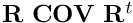. More...

mrpt::math::CVectorDouble getAsVectorVal () const
Return the pose or point as a 1xN vector with all the components (see derived classes for each implementation) More...

mrpt::math::CMatrixDouble44 getHomogeneousMatrixVal () const
Returns the corresponding 4x4 homogeneous transformation matrix for the point(translation) or pose (translation+orientation). More...

void getInverseHomogeneousMatrix (mrpt::math::CMatrixDouble44 &out_HM) const
Returns the corresponding 4x4 inverse homogeneous transformation matrix for this point or pose. More...

mrpt::math::CMatrixDouble44 getInverseHomogeneousMatrix () const

static bool is3DPoseOrPoint ()
Return true for poses or points with a Z component, false otherwise. More...

Member Typedef Documentation

 typedef const double& mrpt::poses::CPose3DQuat::const_reference

Definition at line 251 of file CPose3DQuat.h.

 typedef std::reverse_iterator mrpt::poses::CPose3DQuat::const_reverse_iterator

Definition at line 404 of file CPose3DQuat.h.

 typedef CPose3DQuatPtr mrpt::poses::CPose3DQuat::ConstPtr

Definition at line 44 of file CPose3DQuat.h.

Definition at line 253 of file CPose3DQuat.h.

See ops_containers.h.

Definition at line 423 of file CPose3DQuat.h.

 typedef CPose3DQuatPtr mrpt::poses::CPose3DQuat::Ptr

A typedef for the associated smart pointer

Definition at line 44 of file CPose3DQuat.h.

 typedef double& mrpt::poses::CPose3DQuat::reference

Definition at line 250 of file CPose3DQuat.h.

 typedef std::reverse_iterator mrpt::poses::CPose3DQuat::reverse_iterator

Definition at line 403 of file CPose3DQuat.h.

 typedef std::size_t mrpt::poses::CPose3DQuat::size_type

Definition at line 252 of file CPose3DQuat.h.

Used to emulate CPosePDF types, for example, in mrpt::graphs::CNetworkOfPoses.

Definition at line 237 of file CPose3DQuat.h.

 typedef double mrpt::poses::CPose3DQuat::value_type

The type of the elements.

Definition at line 249 of file CPose3DQuat.h.

Member Enumeration Documentation

 anonymous enum
Enumerator
is_3D_val

Definition at line 238 of file CPose3DQuat.h.

 anonymous enum
Enumerator
rotation_dimensions

Definition at line 240 of file CPose3DQuat.h.

 anonymous enum
Enumerator
is_PDF_val

Definition at line 241 of file CPose3DQuat.h.

 anonymous enum
Enumerator
static_size

Definition at line 256 of file CPose3DQuat.h.

Constructor & Destructor Documentation

 mrpt::poses::CPose3DQuat::CPose3DQuat ( )
inline

Default constructor, initialize translation to zeros and quaternion to no rotation.

Definition at line 63 of file CPose3DQuat.h.

 mrpt::poses::CPose3DQuat::CPose3DQuat ( mrpt::math::TConstructorFlags_Quaternions )
inline

Constructor which left all the quaternion members un-initialized, for use when speed is critical; Use UNINITIALIZED_POSE as argument to this constructor.

Definition at line 66 of file CPose3DQuat.h.

 mrpt::poses::CPose3DQuat::CPose3DQuat ( TConstructorFlags_Poses )
inline

This is an overloaded member function, provided for convenience. It differs from the above function only in what argument(s) it accepts.

Definition at line 68 of file CPose3DQuat.h.

 mrpt::poses::CPose3DQuat::CPose3DQuat ( const double x, const double y, const double z, const mrpt::math::CQuaternionDouble & q )
inline

Constructor with initilization of the pose - the quaternion is normalized to make sure it's unitary.

Definition at line 71 of file CPose3DQuat.h.

 CPose3DQuat::CPose3DQuat ( const CPose3D & p )
explicit

Constructor from a CPose3D.

Definition at line 27 of file CPose3DQuat.cpp.

 mrpt::poses::CPose3DQuat::CPose3DQuat ( const mrpt::math::TPose3DQuat & p )
inline

Constructor from lightweight object.

Definition at line 77 of file CPose3DQuat.h.

 CPose3DQuat::CPose3DQuat ( const mrpt::math::CMatrixDouble44 & M )
explicit

Constructor from a 4x4 homogeneous transformation matrix.

Definition at line 37 of file CPose3DQuat.cpp.

References mrpt::poses::CPose3D::getAsQuaternion(), m_coords, and m_quat.

Member Function Documentation

 static const mrpt::utils::TRuntimeClassId* mrpt::poses::CPose3DQuat::_GetBaseClass ( )
staticprotected
 void mrpt::poses::CPose3DQuat::assign ( const size_t N, const double val )
inline

Definition at line 262 of file CPose3DQuat.h.

 void mrpt::poses::CPose3DQuat::asString ( std::string & s ) const
inline

Returns a human-readable textual representation of the object (eg: "[x y z qr qx qy qz]", angles in degrees.)

fromString

Definition at line 175 of file CPose3DQuat.h.

References mrpt::mrpt::format().

Referenced by mrpt::utils::TStereoCamera::saveToConfigFile().

 std::string mrpt::poses::CPose3DQuat::asString ( ) const
inline

Definition at line 176 of file CPose3DQuat.h.

References asString().

Referenced by asString().

 iterator mrpt::poses::CPose3DQuat::begin ( )
inline

Definition at line 405 of file CPose3DQuat.h.

 const_iterator mrpt::poses::CPose3DQuat::begin ( ) const
inline

Definition at line 407 of file CPose3DQuat.h.

 CObject* mrpt::utils::CObject::clone ( ) const
inlineinherited

Cloning interface for smart pointers.

Definition at line 143 of file CObject.h.

 void CPose3DQuat::composeFrom ( const CPose3DQuat & A, const CPose3DQuat & B )

Makesthis method is slightly more efficient than "this= A + B;" since it avoids the temporary object.

Makes "this = A (+) B"; this method is slightly more efficient than "this= A + B;" since it avoids the temporary object.

Note
A or B can be "this" without problems.
inverseComposeFrom, composePoint
Note
A or B can be "this" without problems.

Definition at line 75 of file CPose3DQuat.cpp.

Referenced by operator+().

 void CPose3DQuat::composePoint ( const double lx, const double ly, const double lz, double & gx, double & gy, double & gz, mrpt::math::CMatrixFixedNumeric< double, 3, 3 > * out_jacobian_df_dpoint = NULL, mrpt::math::CMatrixFixedNumeric< double, 3, 7 > * out_jacobian_df_dpose = NULL ) const

Computes the 3D point G such as.

composeFrom, inverseComposePoint
inverseComposeFrom

Definition at line 105 of file CPose3DQuat.cpp.

template<class POINT1 , class POINT2 >
 void mrpt::poses::CPose3DQuat::composePoint ( const POINT1 & L, POINT2 & G ) const
inline

Computes the 3D point G such as.

POINT1 and POINT1 can be anything supporing ,,.

composePoint

Definition at line 125 of file CPose3DQuat.h.

References composePoint().

Referenced by composePoint().

 static CPose3DQuatPtr mrpt::poses::CPose3DQuat::Create ( )
static
 static mrpt::utils::CObject* mrpt::poses::CPose3DQuat::CreateObject ( )
static
 double mrpt::poses::CPoseOrPoint< CPose3DQuat >::distance2DTo ( double ax, double ay ) const
inlineinherited

Returns the 2D distance from this pose/point to a 2D point (ignores Z, if it exists).

Definition at line 165 of file CPoseOrPoint.h.

 double mrpt::poses::CPoseOrPoint< CPose3DQuat >::distance2DToSquare ( double ax, double ay ) const
inlineinherited

Returns the squared 2D distance from this pose/point to a 2D point (ignores Z, if it exists).

Definition at line 156 of file CPoseOrPoint.h.

 double mrpt::poses::CPoseOrPoint< CPose3DQuat >::distance3DTo ( double ax, double ay, double az ) const
inlineinherited

Returns the 3D distance from this pose/point to a 3D point.

Definition at line 168 of file CPoseOrPoint.h.

 double mrpt::poses::CPoseOrPoint< CPose3DQuat >::distance3DToSquare ( double ax, double ay, double az ) const
inlineinherited

Returns the squared 3D distance from this pose/point to a 3D point.

Definition at line 159 of file CPoseOrPoint.h.

 double mrpt::poses::CPoseOrPoint< CPose3DQuat >::distanceTo ( const CPoseOrPoint< OTHERCLASS > & b ) const
inlineinherited

Returns the Euclidean distance to another pose/point:

Definition at line 150 of file CPoseOrPoint.h.

 double mrpt::poses::CPoseOrPoint< CPose3DQuat >::distanceTo ( const mrpt::math::TPoint3D & b ) const
inlineinherited

Returns the euclidean distance to a 3D point:

Definition at line 171 of file CPoseOrPoint.h.

 virtual mrpt::utils::CObject* mrpt::poses::CPose3DQuat::duplicate ( ) const
virtual

Returns a copy of the object, indepently of its class.

Implements mrpt::utils::CObject.

 mrpt::utils::CObjectPtr mrpt::utils::CObject::duplicateGetSmartPtr ( ) const
inlineinherited

Returns a copy of the object, indepently of its class, as a smart pointer (the newly created object will exist as long as any copy of this smart pointer).

Definition at line 140 of file CObject.h.

 static bool mrpt::poses::CPose3DQuat::empty ( )
inlinestatic

Definition at line 258 of file CPose3DQuat.h.

 iterator mrpt::poses::CPose3DQuat::end ( )
inline

Definition at line 406 of file CPose3DQuat.h.

References static_size.

 const_iterator mrpt::poses::CPose3DQuat::end ( ) const
inline

Definition at line 408 of file CPose3DQuat.h.

References static_size.

 void mrpt::poses::CPose3DQuat::fromString ( const std::string & s )
inline

Set the current object value from a string generated by 'asString' (eg: "[0.02 1.04 -0.8 1 0 0 0]" )

asString
Exceptions
 std::exception On invalid format

Definition at line 182 of file CPose3DQuat.h.

References ASSERTMSG_, mrpt::mrpt::math::size(), and THROW_EXCEPTION.

 void CPose3DQuat::getAsVector ( mrpt::math::CVectorDouble & v ) const

Returns a 1x7 vector with [x y z qr qx qy qz].

Definition at line 60 of file CPose3DQuat.cpp.

References m_coords, and m_quat.

 void mrpt::poses::CPose3DQuat::getAsVector ( mrpt::math::CArrayDouble< 7 > & v ) const
inline

This is an overloaded member function, provided for convenience. It differs from the above function only in what argument(s) it accepts.

Definition at line 91 of file CPose3DQuat.h.

 mrpt::math::CVectorDouble mrpt::poses::CPoseOrPoint< CPose3DQuat >::getAsVectorVal ( ) const
inlineinherited

Return the pose or point as a 1xN vector with all the components (see derived classes for each implementation)

Definition at line 181 of file CPoseOrPoint.h.

 void CPose3DQuat::getHomogeneousMatrix ( mrpt::math::CMatrixDouble44 & out_HM ) const

Returns the corresponding 4x4 homogeneous transformation matrix for the point(translation) or pose (translation+orientation).

getInverseHomogeneousMatrix

Definition at line 49 of file CPose3DQuat.cpp.

References m_coords, m_quat, and mrpt::math::CQuaternion< T >::rotationMatrixNoResize().

 mrpt::math::CMatrixDouble44 mrpt::poses::CPoseOrPoint< CPose3DQuat >::getHomogeneousMatrixVal ( ) const
inlineinherited

Returns the corresponding 4x4 homogeneous transformation matrix for the point(translation) or pose (translation+orientation).

getInverseHomogeneousMatrix

Definition at line 191 of file CPoseOrPoint.h.

 void mrpt::poses::CPoseOrPoint< CPose3DQuat >::getInverseHomogeneousMatrix ( mrpt::math::CMatrixDouble44 & out_HM ) const
inlineinherited

Returns the corresponding 4x4 inverse homogeneous transformation matrix for this point or pose.

getHomogeneousMatrix

Definition at line 201 of file CPoseOrPoint.h.

 mrpt::math::CMatrixDouble44 mrpt::poses::CPoseOrPoint< CPose3DQuat >::getInverseHomogeneousMatrix ( ) const
inlineinherited

This is an overloaded member function, provided for convenience. It differs from the above function only in what argument(s) it accepts.

Definition at line 208 of file CPoseOrPoint.h.

 const type_value& mrpt::poses::CPose3DQuat::getPoseMean ( ) const
inline

Definition at line 244 of file CPose3DQuat.h.

 type_value& mrpt::poses::CPose3DQuat::getPoseMean ( )
inline

Definition at line 245 of file CPose3DQuat.h.

 virtual const mrpt::utils::TRuntimeClassId* mrpt::poses::CPose3DQuat::GetRuntimeClass ( ) const
virtual

Returns information about the class of an object in runtime.

Reimplemented from mrpt::utils::CSerializable.

 void CPose3DQuat::inverse ( )

Convert this pose into its inverse, saving the result in itself.

operator-

Definition at line 432 of file CPose3DQuat.cpp.

References inverseComposePoint(), m_coords, and m_quat.

Referenced by mrpt::poses::operator-().

 void CPose3DQuat::inverseComposeFrom ( const CPose3DQuat & A, const CPose3DQuat & B )

Makesthis method is slightly more efficient than "this= A - B;" since it avoids the temporary object.

Note
A or B can be "this" without problems.
composeFrom, composePoint
Note
A or B can be "this" without problems.
composeFrom

Definition at line 92 of file CPose3DQuat.cpp.

Referenced by operator-().

 void CPose3DQuat::inverseComposePoint ( const double gx, const double gy, const double gz, double & lx, double & ly, double & lz, mrpt::math::CMatrixFixedNumeric< double, 3, 3 > * out_jacobian_df_dpoint = NULL, mrpt::math::CMatrixFixedNumeric< double, 3, 7 > * out_jacobian_df_dpose = NULL ) const

Computes the 3D point L such as.

Computes the 3D point G such as.

composePoint, composeFrom
composeFrom

Definition at line 181 of file CPose3DQuat.cpp.

template<class POINT1 , class POINT2 >
 void mrpt::poses::CPose3DQuat::inverseComposePoint ( const POINT1 & G, POINT2 & L ) const
inline

Computes the 3D point L such as.

inverseComposePoint

Definition at line 128 of file CPose3DQuat.h.

References inverseComposePoint().

Referenced by inverseComposePoint().

 static bool mrpt::poses::CPoseOrPoint< CPose3DQuat >::is3DPoseOrPoint ( )
inlinestaticinherited

Return true for poses or points with a Z component, false otherwise.

Definition at line 127 of file CPoseOrPoint.h.

 static bool mrpt::poses::CPose3DQuat::is_3D ( )
inlinestatic

Definition at line 239 of file CPose3DQuat.h.

 static bool mrpt::poses::CPose3DQuat::is_PDF ( )
inlinestatic

Definition at line 242 of file CPose3DQuat.h.

 static size_type mrpt::poses::CPose3DQuat::max_size ( )
inlinestatic

Definition at line 259 of file CPose3DQuat.h.

References static_size.

 double mrpt::poses::CPoseOrPoint< CPose3DQuat >::norm ( ) const
inlineinherited

Returns the euclidean norm of vector:.

Definition at line 174 of file CPoseOrPoint.h.

 void mrpt::poses::CPose3DQuat::operator delete ( void * ptr ) throw ( )
inline

Definition at line 44 of file CPose3DQuat.h.

 void mrpt::poses::CPose3DQuat::operator delete ( void * memory, void * ptr ) throw ( )
inline

Definition at line 44 of file CPose3DQuat.h.

 void mrpt::poses::CPose3DQuat::operator delete ( void * ptr, const std::nothrow_t & ) throw ( )
inline

Definition at line 44 of file CPose3DQuat.h.

 void mrpt::poses::CPose3DQuat::operator delete[] ( void * ptr ) throw ( )
inline

Definition at line 44 of file CPose3DQuat.h.

 void* mrpt::poses::CPose3DQuat::operator new ( size_t size, const std::nothrow_t & ) throw ( )
inline

Definition at line 44 of file CPose3DQuat.h.

 static void* mrpt::poses::CPose3DQuat::operator new ( size_t size, void * ptr )
inlinestatic

Definition at line 44 of file CPose3DQuat.h.

 void* mrpt::poses::CPose3DQuat::operator new ( size_t size )
inline

Definition at line 44 of file CPose3DQuat.h.

 void* mrpt::poses::CPose3DQuat::operator new[] ( size_t size )
inline

Definition at line 44 of file CPose3DQuat.h.

 void CPose3DQuat::operator*= ( const double s )
virtual

Scalar multiplication (all x y z qr qx qy qz elements are multiplied by the scalar).

Definition at line 286 of file CPose3DQuat.cpp.

References m_coords, and m_quat.

 CPoint3D mrpt::poses::CPose3DQuat::operator+ ( const CPoint3D & L ) const
inline

Computes the 3D point G such as.

composePoint

Definition at line 131 of file CPose3DQuat.h.

 mrpt::math::TPoint3D mrpt::poses::CPose3DQuat::operator+ ( const mrpt::math::TPoint3D & L ) const
inline

Computes the 3D point G such as.

composePoint

Definition at line 134 of file CPose3DQuat.h.

 CPose3DQuat mrpt::poses::CPose3DQuat::operator+ ( const CPose3DQuat & p ) const
inline

Return the composed pose.

Definition at line 147 of file CPose3DQuat.h.

References composeFrom().

 CPose3DQuat& mrpt::poses::CPose3DQuat::operator+= ( const CPose3DQuat & b )
inline

Make.

Definition at line 140 of file CPose3DQuat.h.

 CPose3DQuat mrpt::poses::CPose3DQuat::operator- ( const CPose3DQuat & p ) const
inline

Return the composed pose.

Definition at line 162 of file CPose3DQuat.h.

References inverseComposeFrom().

 CPose3DQuat& mrpt::poses::CPose3DQuat::operator-= ( const CPose3DQuat & b )
inline

Make.

Definition at line 155 of file CPose3DQuat.h.

 const double& mrpt::poses::CPose3DQuat::operator[] ( unsigned int i ) const
inline

Definition at line 191 of file CPose3DQuat.h.

 double& mrpt::poses::CPose3DQuat::operator[] ( unsigned int i )
inline

Definition at line 207 of file CPose3DQuat.h.

 mrpt::math::CQuaternionDouble& mrpt::poses::CPose3DQuat::quat ( )
inline
 const mrpt::math::CQuaternionDouble& mrpt::poses::CPose3DQuat::quat ( ) const
inline

Definition at line 54 of file CPose3DQuat.h.

 reverse_iterator mrpt::poses::CPose3DQuat::rbegin ( )
inline

Definition at line 409 of file CPose3DQuat.h.

 const_reverse_iterator mrpt::poses::CPose3DQuat::rbegin ( ) const
inline

Definition at line 410 of file CPose3DQuat.h.

 void CPose3DQuat::readFromStream ( mrpt::utils::CStream & in, int version )
protectedvirtual

Introduces a pure virtual method responsible for loading from a CStream This can not be used directly be users, instead use "stream >> object;" for reading it from a stream or "stream >> object_ptr;" if the class is unknown apriori.

Parameters
 in The input binary stream where the object data must read from. version The version of the object stored in the stream: use this version number in your code to know how to read the incoming data.
Exceptions
 std::exception On any error, see CStream::ReadBuffer
CStream

Implements mrpt::utils::CSerializable.

Definition at line 315 of file CPose3DQuat.cpp.

References m_coords, m_quat, and MRPT_THROW_UNKNOWN_SERIALIZATION_VERSION.

 reverse_iterator mrpt::poses::CPose3DQuat::rend ( )
inline

Definition at line 411 of file CPose3DQuat.h.

References begin().

 const_reverse_iterator mrpt::poses::CPose3DQuat::rend ( ) const
inline

Definition at line 412 of file CPose3DQuat.h.

References begin().

 static void mrpt::poses::CPose3DQuat::resize ( const size_t n )
inlinestatic

Definition at line 260 of file CPose3DQuat.h.

References mrpt::format(), and static_size.

 void CPose3DQuat::setToNaN ( )
virtual

Set all data fields to quiet NaN.

Implements mrpt::poses::CPoseOrPoint< CPose3DQuat >.

Definition at line 445 of file CPose3DQuat.cpp.

References m_coords, and quat().

 static size_type mrpt::poses::CPose3DQuat::size ( )
inlinestatic

Definition at line 257 of file CPose3DQuat.h.

References static_size.

Referenced by mrpt::slam::CRangeBearingKFSLAM::OnObservationModel().

 void CPose3DQuat::sphericalCoordinates ( const mrpt::math::TPoint3D & point, double & out_range, double & out_yaw, double & out_pitch, mrpt::math::CMatrixFixedNumeric< double, 3, 3 > * out_jacob_dryp_dpoint = NULL, mrpt::math::CMatrixFixedNumeric< double, 3, 7 > * out_jacob_dryp_dpose = NULL ) const

Computes the spherical coordinates of a 3D point as seen from the 6D pose specified by this object.

For the coordinate system see the top of this page. If the matrix pointers are not NULL, the Jacobians will be also computed for the range-yaw-pitch variables wrt the passed 3D point and this 7D pose.

Definition at line 332 of file CPose3DQuat.cpp.

 double mrpt::poses::CPoseOrPoint< CPose3DQuat >::sqrDistanceTo ( const CPoseOrPoint< OTHERCLASS > & b ) const
inlineinherited

Returns the squared euclidean distance to another pose/point:

Definition at line 130 of file CPoseOrPoint.h.

 void mrpt::poses::CPose3DQuat::swap ( CPose3DQuat & o )
inline

Definition at line 415 of file CPose3DQuat.h.

References m_coords, and m_quat.

 virtual mxArray* mrpt::utils::CSerializable::writeToMatlab ( ) const
inlinevirtualinherited

Introduces a pure virtual method responsible for writing to a mxArray Matlab object, typically a MATLAB struct whose contents are documented in each derived class.

Returns
A new mxArray (caller is responsible of memory freeing) or NULL is class does not support conversion to MATLAB.

Definition at line 79 of file CSerializable.h.

 void CPose3DQuat::writeToStream ( mrpt::utils::CStream & out, int * getVersion ) const
protectedvirtual

Introduces a pure virtual method responsible for writing to a CStream.

This can not be used directly be users, instead use "stream << object;" for writing it to a stream.

Parameters
 out The output binary stream where object must be dumped. getVersion If NULL, the object must be dumped. If not, only the version of the object dump must be returned in this pointer. This enables the versioning of objects dumping and backward compatibility with previously stored data.
Exceptions
 std::exception On any error, see CStream::WriteBuffer
CStream

Implements mrpt::utils::CSerializable.

Definition at line 301 of file CPose3DQuat.cpp.

References m_coords, and m_quat.

 double mrpt::poses::CPoseOrPoint< CPose3DQuat >::x ( ) const
inlineinherited

Common members of all points & poses classes.

< Get X coord.

Definition at line 113 of file CPoseOrPoint.h.

 double& mrpt::poses::CPoseOrPoint< CPose3DQuat >::x ( )
inlineinherited

Definition at line 116 of file CPoseOrPoint.h.

 void mrpt::poses::CPoseOrPoint< CPose3DQuat >::x ( const double v )
inlineinherited
Parameters
 v Set X coord.

Definition at line 119 of file CPoseOrPoint.h.

 void mrpt::poses::CPoseOrPoint< CPose3DQuat >::x_incr ( const double v )
inlineinherited
Parameters
 v X+=v

Definition at line 122 of file CPoseOrPoint.h.

 mrpt::math::CArrayDouble<3>& mrpt::poses::CPose3DQuat::xyz ( )
inline

Definition at line 57 of file CPose3DQuat.h.

 const mrpt::math::CArrayDouble<3>& mrpt::poses::CPose3DQuat::xyz ( ) const
inline

Definition at line 59 of file CPose3DQuat.h.

 double mrpt::poses::CPoseOrPoint< CPose3DQuat >::y ( ) const
inlineinherited

< Get Y coord.

Definition at line 114 of file CPoseOrPoint.h.

 double& mrpt::poses::CPoseOrPoint< CPose3DQuat >::y ( )
inlineinherited

Definition at line 117 of file CPoseOrPoint.h.

 void mrpt::poses::CPoseOrPoint< CPose3DQuat >::y ( const double v )
inlineinherited
Parameters
 v Set Y coord.

Definition at line 120 of file CPoseOrPoint.h.

 void mrpt::poses::CPoseOrPoint< CPose3DQuat >::y_incr ( const double v )
inlineinherited
Parameters
 v Y+=v

Definition at line 123 of file CPoseOrPoint.h.

Member Data Documentation

 mrpt::utils::CLASSINIT mrpt::poses::CPose3DQuat::_init_CPose3DQuat
staticprotected

Definition at line 44 of file CPose3DQuat.h.

 const mrpt::utils::TRuntimeClassId mrpt::utils::CObject::classCObject
staticinherited

Definition at line 128 of file CObject.h.

 mrpt::utils::TRuntimeClassId mrpt::poses::CPose3DQuat::classCPose3DQuat
static

Definition at line 44 of file CPose3DQuat.h.

 const mrpt::utils::TRuntimeClassId mrpt::utils::CSerializable::classCSerializable
staticinherited

Definition at line 42 of file CSerializable.h.

 const mrpt::utils::TRuntimeClassId* mrpt::poses::CPose3DQuat::classinfo
static

Definition at line 44 of file CPose3DQuat.h.

 mrpt::math::CArrayDouble<3> mrpt::poses::CPose3DQuat::m_coords

 Page generated by Doxygen 1.8.6 for MRPT 1.5.6 Git: 4c65e84 Tue Apr 24 08:18:17 2018 +0200 at mar abr 24 08:26:17 CEST 2018